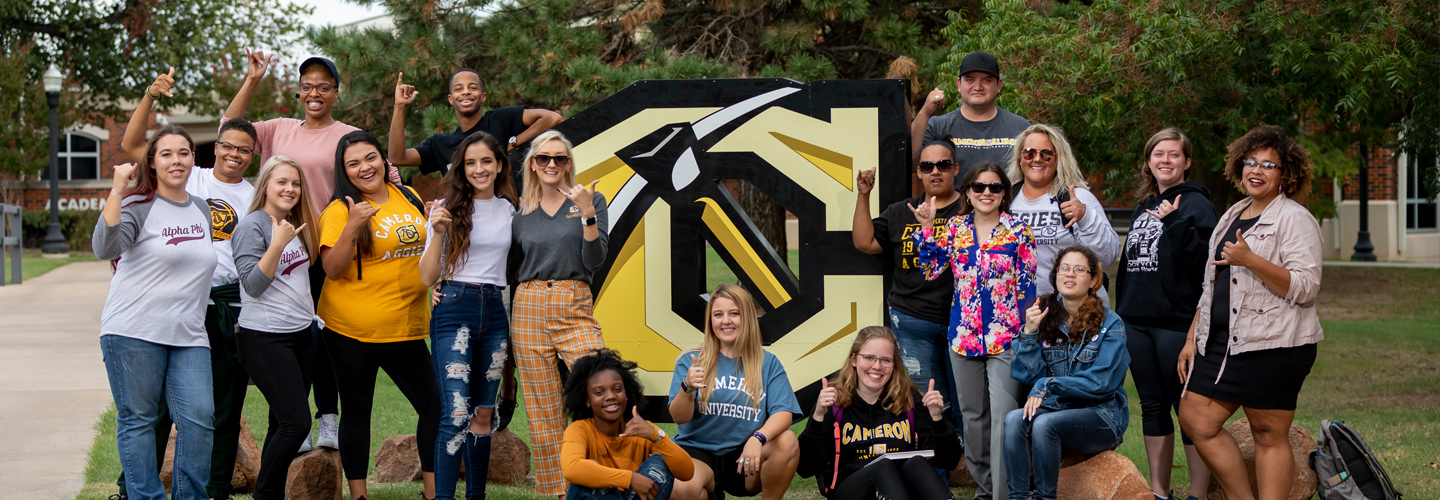# PROGRAMS IN THE DEPARTMENT OF COMPUTING AND Mathematical Sciences

### 150 - Mathematics - BA Outcomes

1. Students will demonstrate a conceptual understanding of algebra, geometry, trigonometry, and calculus concepts.
2. Students will apply major concepts from advanced algebra.
3. Students will analyze ordinary differential equations.
4. Students will construct mathematically rigorous and logically correct proofs.
5. Students will formulate mathematical models that arise in real-world situations.
6. Students will utilize technology as an effective tool in investigating, understanding, and applying mathematics.
7. Students will analyze and solve problems through the application of concepts from algebra, geometry, trigonometry, and calculus.

### 214 Certificate in Cyber-Security

1. Students will recognize IT ethical issues.
2. Students will effectively communicate in writing with people in the information technology field.
3. Students will demonstrate proficiency at computer network installation.
4. Students will effectively communicate orally with people in the information technology field.

### 414 - Information Technology - BS Outcomes

1. Students will analyze IT ethical issues.
2. Students will effectively communicate in writing with people in the information technology field.
3. Students will develop computer programs using a high level programming language.
4. Students will demonstrate proficiency at computer network installation.
5. Students will demonstrate proficiency at database development.
6. Students will demonstrate an ability to work effectively in teams.
7. Students will effectively communicate orally with people in the information technology field.

### 415 - Computer Science - BS Outcomes

1. Students will design and implement a working computer program that solves a specific problem.
2. Students will apply data structures and efficient algorithms to solve problems.
3. Students will solve problems related to operating systems and networking.
4. Students will explain software solutions effectively.

### 514 - Information Technology - AAS Outcomes

1. Students will analyze IT ethical issues.
2. Students will effectively communicate in writing with people in the information technology field.
3. Students will develop computer programs using a programming language.
4. Students will demonstrate proficiency at computer network installation.
5. Students will effectively communicate orally with people in the information technology field.